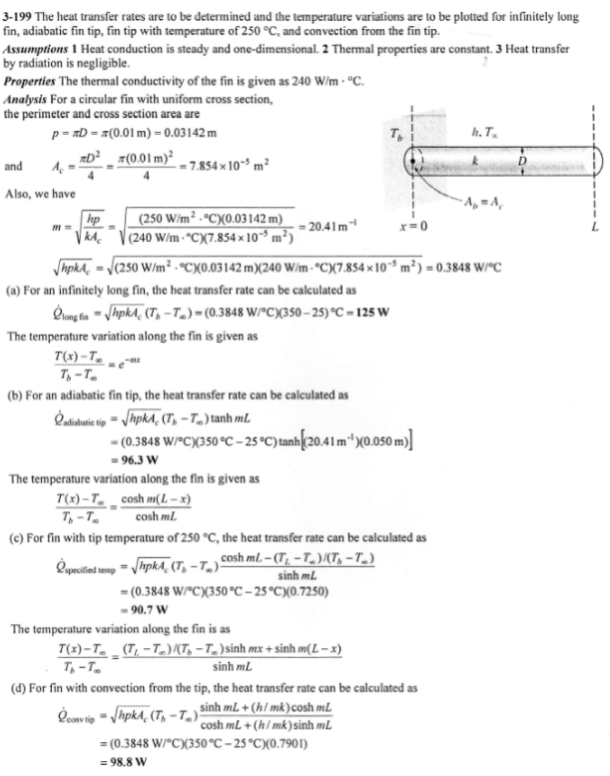heat and mass transfer problems engineering equations heat and mass transfer school homework engineering heat and mass transfer formulas heat and mass transfer solutions to heat and mass transfer problems full solution engineering problem solution heat and mass transfer math problems engineering equations heat and mass transfer school homework engineering solutions to heat and mass transfer formulas heat problems mass problem solutions to transfer problems full solution heat and mass transfer
heat and mass transfer problems engineering equations heat and mass transfer school homework engineering heat and mass transfer formulas heat and mass transfer solutions to heat and mass transfer problems full solution engineering problem solution heat and mass transfer math problems engineering equations heat and mass transfer school homework engineering solutions to heat and mass transfer formulas heat problems mass problem solutions to transfer problems full solution heat and mass transfer
Highalphabet Home Page heat and mass transfer problem solutions Heat and Mass Transfer Page
Circular fins of uniform cross section, with diameter of 10 mm and length of 50 mm, are attached to a wall with surface temperature of 350 C. The fins are made of material with thermal conductivity of 240 W/mK, and they are exposed to an ambient air condition of 25 C and the convection heat transfer coefficient is 250 W/m^2 K. Determine the heat transfer rate and plot the temperature variation of a single fin for the following boundary conditions: (a) infinitely long fin (b) Adiabatic fin tip (c) fin with tip temperature of 250 C (d) convection from the fin tip.Circular fins of uniform cross section, with diameter of 10 mm and length of 50 mm, are attached to a wall with surface temperature of 350 C. The fins are made of material with thermal conductivity of 240 W/mK, and they are exposed to an ambient air condition of 25 C and the convection heat transfer coefficient is 250 W/m^2 K. Determine the heat transfer rate and plot the temperature variation of a single fin for the following boundary conditions: (a) infinitely long fin (b) Adiabatic fin tip (c) fin with tip temperature of 250 C (d) convection from the fin tip.